# We Helped With This R Studio Programming Assignment: Have A Similar One?

SOLVED## Assignment Description

GEOG 5670 Spatial Analysis                                                                                 Name:  __________________

Homework # 4

Regression

1.       Simple regression

a.       Write the regression equation for this simple model

b.       Compute the mean of the BalHouses\$price column of your dataframe using the mean() function.  How does this compare to the intercept from your regression equation

c.       Compute the standard deviation of BalHouses\$price.  How does this compare to the standard error of your simple regression model?

2.       Full model

a.       How many dummy variables did the lm() function create for the bment categorical variable? List what values each of these would have for the four different basement types

b.       Write the regression equation for the complex model

c.       What is the effect on the estimated price for a house with a full unfinished basement as compared to an identical one having a full finished basement?

d.       What is the R2 for this model?  What does this represent?

e.       Which of the independent variables are not significant at the p = 0.05 level?

f.        Plug the following values for the independent variables into the regression equation to estimate a price:

g.  Check for collinearity in this model by using the vif() function on BalFull (assume VIF > 5 is problematic).  List which (if any) variables exhibit multicollinearity.

3.       Stepwise variable entry

a.       What were the starting and ending AIC values?

b.       What was the final regression equation?

c.       Which has a greater impact on the predicted price, adding another bathroom, or adding air conditioning?

d.       What is the R2 for this model?  How does this compare to the full model from question #2?

e.       Check for collinearity in this model by using the vif() function on BalStepwise.  How do these values compare to those from Question 2f?

f.        Paste diagnostic plots here

g.       By examining the values for the dependent and independent variables for the Balhouses outlier, what do you think would account for the large residual?  Did the model underestimate or overestimate the price for this house?

h.       Residuals vs. Leverage plot.  How many houses are definitely outside this range?  Cook’s distance critical values are printed as dashed lines for values of moderate concern (0.5) and serious concern (1.0).  Are there any samples near or exceeding these values?

i.         Sample #20 in the BalHouses table. What is unusual about this house than makes it have so much leverage?

j.         Using the example independent variable values from question 2f recompute the estimated price for that house.  How does this compare to the full model’s estimate?

4.       Forward and Backward variable selection

a.       How do these final models compare to the final model built by stepwise selection?

## Assignment Description

GEOG 5670 Spatial Analysis

Dr. Emerson                                                        Homework # 4

Regression

The Baltimore Realtor’s Association has compiled a database of 211 home prices with some basic descriptive attributes about each.

 Field Description price Price ( x \$1,000) nroom Number of rooms nbath Number of bathrooms ac Is home air conditioned (1 = yes, 0 = no) bment Basement description (None, Partial, Full Unfinished, Full Finished) gar Number of enclosed spaces to park a car age Age of home in years lotsz Size of lot (x 100 sq. ft.) sqft Size of home interior (x 100 sq. ft.)

This data is contained in the Baltimore.csv comma delimited text file on the GEOG 5670 Elearning page.  Copy this file to a folder on your USB drive called Regression.  Navigate to this folder in RStudio, make this the working directory, and import the data into an object called BalHouses using the read.csv() function.  You will also need to load the car package to get some of the diagnostic tools.  Answer the following questions in a Word document to turn in (note:  you’ll be pasting in some plots later on).

1.       Run a simple regression on the housing data using the lm() function.  We’ll initially assume the best prediction of housing prices is simply the mean, so specify the model as price ~ 1   and save the output of lm() as an object titled, BalNull.  Use the summary(BalNull) function to get information for this model.

a.       Write the regression equation for this simple model

b.       Compute the mean of the BalHouses\$price column of your dataframe using the mean() function.  How does this compare to the intercept from your regression equation

c.       Compute the standard deviation of BalHouses\$price.  How does this compare to the standard error of your simple regression model?

2.       Now run the lm() function with all of the predictor variables entered.  Save the result into an object called BalFull. Note that since the bment variable is a categorical factor, lm() automatically turns it into dummy variables.  Use the summary(BalFull) function to get information for this model.  Run the summary() function on the BalHouses dataframe to get some basic descriptive statistics for the input data.

a.       How many dummy variables did the lm() function create for the bment categorical variable?

List what values each of these would have for the four different basement types

b.       Write the regression equation for the complex model

c.       What is the effect on the estimated price for a house with a full unfinished basement as compared to an identical one having a full finished basement?

d.       What is the R2 for this model?  What does this represent?

e.       Which of the independent variables are not significant at the p = 0.05 level?

f.        Plug the following values for the independent variables into the regression equation and use a calculator to estimate a price:

 Variable Description nroom 6 nbath 2 ac Yes (use a value of 1) bment Choose the appropriate values for the dummy variables for a Full Unfinished basement gar 2 age 25 lotsz 75 sqft 18

g.       Check for collinearity in this model by using the vif() function on BalFull (assume VIF > 5 is problematic).  List which (if any) variables exhibit multicollinearity.

Since we’re just exploring the dataset at this point, we’ll try stepwise variable selection to see which combination of the independent variables are good predictors of price.

3.       Use the step() function on the BalNull object you generated in question #1 to do stepwise variable selection.  Save the results of step() to an object titled BalStepwise.  Set the scope to the full model:

price ~ nroom + nbath + ac + bment + gar + age + lotsz + sqft  and specify direction = “both” .

a.       What were the starting and ending AIC values?

b.       What was the final regression equation?

c.       Which has a greater impact on the predicted price, adding another bathroom, or adding air conditioning?

d.       What is the R2 for this model?  How does this compare to the full model from question #2?

e.       Check for collinearity in this model by using the vif() function on BalStepwise.  How do these values compare to those from Question 2f?

f.        In the console window, use the plot() function on BalStepwise to generate some diagnostic plots.  You will be prompted to hit <enter> several times, and you can scroll through the plots using the arrows in the plot window.  Copy each of these and paste them into answer sheet along with the answers to the following questions.  It will also be helpful to have the BalHouses dataframe displayed in the upper left pane of RStudio, so click twice on the dataframe name in the Environment window.

g.       In the Residuals vs. Fitted plot, some of the more extreme outliers are identified by their number.  Look at the row in BalHouses corresponding to the most extreme outlier’s number.  By examining the values for the dependent and independent variables for this outlier, what do you think would account for the large residual?  (It may be helpful to refer to the basic descriptive statistics generated earlier from the summary(BalHouses) function. Did the model underestimate or overestimate the price for this house?

h.       Look at the Residuals vs. Leverage plot.  Standardized residuals with values > 3 or < -3 are good candidates for being labeled outliers.  How many houses are definitely outside this range?  Cook’s distance critical values are printed as dashed lines for values of moderate concern (0.5) and serious concern (1.0).  Are there any samples near or exceeding these values?

i.         Look at the values for the independent variables for sample #20 in the BalHouses table. What is unusual about this house than makes it have so much leverage? (note: you may have to look at the basic descriptive statistics generated in Question #2).

j.         Using the example independent variable values from question 2f recompute the estimated price for that house.  How does this compare to the full model’s estimate?

4.       Finally, run a forward variable selection on the BalNull simple model using the same scope as the stepwise selection, except specify “forward” instead of “both”.  Do a backward selection on the BalFull model (note: you don’t have to specify a scope because you are starting with the full model, but you do have to set the direction as “backward”).

a.       How do these final models compare to the final model built by stepwise selection?

## Assignment DescriptionMULTIPLE REGRESSION

Spatial Analysis.

Bivariate vs. Multivariate Regression

Phenomena with only one independent variable are rare

Most often there are many predictors for some outcome

Multiple linear regression model:

Y = b0 + b1 X1 + b2 X 2 +L+ bk Xk + e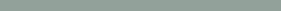Graphical Representation of a Multivariate Relationship

Example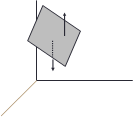Student GPA Verbal Quant High School GPA 1 3.54 580 720 3.82 2 2.62 500 660 2.67 3 3.30 670 580 3.16 4 2.90 480 520 3.31 5 4.00 710 630 3.60 6 3.21 550 690 3.42 7 3.57 640 700 3.51 8 3.05 540 530 2.75 9 3.15 620 490 3.21 10 3.61 690 530 3.70

Y

e(positive)

Y = b0 + b1 X1 + b2 X 2

e(negative)

X1

X2Least Squares Estimates

Estimate b ’s that minimize sum of squares of the

residuals                                      min å(Y - Yˆ)2

Yˆ = b0 + b1 X1 + b2 X 2 +L+ bk Xk

### R Results

Yˆ = -0.395 + 0.003X1 + 0.001X 2 + 0.446X3

Call: lm(formula = GPA ~ Verbal + Quant + HS, data = Undergrad)

Residuals:

Min      1Q     Median  3Q      Max

-0.13457 -0.09841 -0.01565 0.01961 0.23104

Coefficients:

Estimate Std.Error t value Pr(>|t|) (Intercept) -0.3949535 0.5813469 -0.679 0.52223

 Verbal 0.0031028 0.0007987 3.885 0.00813 ** Quant 0.0005900 0.0006699 0.881 0.41232 •  b0, b1 , … bk are the least squares estimates of HS--- 0.4457044 0.1763620 2.527 0.04485 *

b0, b1 , ... bk

Signif. codes: 0 ‘***’ 0.001 ‘**’ 0.01 ‘*’ 0.05 ‘.’ 0.1 ‘ ’ 1 Residual standard error: 0.1594 on 6 degrees of freedom Multiple R-squared: 0.8935, Adjusted R-squared: 0.8403

F-statistic: 16.78 on 3 and 6 DF, p-value: 0.002532

### Interpreting Regression Coefficients

#### • This is only true if the independent variables are

unrelated

Assumptions

Same as for bivariate least squares regression

Errors follow a normal distribution, centered at zero

Homoscedasticity of errors (variance is constant)

Errors are statistically independent

Yˆ = -0.395 + 0.003X1 + 0.001X 2 + 0.446X3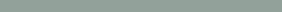### Residual Standard Deviation

##### • For n samples and k independent variables,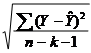s =

##### • If s2 = 0, then SSE = 0 and Y = Ŷ

Hypothesis Test

Ho: b1 = b2 = … = bk = 0

##### • Ha: at least one of the b’s ≠ 0

Rejecting Ho means that at least one (but not necessarily all) of the independent variables contribute significantly to the prediction of Y

Failing to reject Ho means that we can’t use this set of independent variables to explain the dependentHypothesis Test and Confidence Intervals for Independent Variables

Estimate Std.Error t value Pr(>|t|) (Intercept) -0.3949535 0.5813469 -0.679 0.52223

### Warning

Dropping an independent variable from a regression model does not mean we can keep the same coefficients for the remaining variables

Yˆ = -0.395 + 0.003X1 + 0.001X 2 + 0.446 X3 ¹ -0.395 + 0.003X1 + 0.446 X3

X1 and X3 are related, so we must recompute the regression

 Verbal 0.0031028 0.0007987 3.885 0.00813 ** Quant 0.0005900 0.0006699 0.881 0.41232 •  Ho: bi = 0 HS 0.4457044 0.1763620 2.527 0.04485 *

Ha: bi 0

Test statisticb

t =  bi s

i

tcrit

= t0.05,10-3-1=6

= 1.943

Call: lm(formula = GPA ~ Verbal + HS, data = Undergrad) Residuals:

Min         1Q       Median    3Q        Max

-0.15701 -0.11239 -0.02073 0.05103 0.23195

Coefficients:

Estimate Std. Error t value Pr(>|t|) (Intercept) -0.1320967 0.4908430 -0.269 0.79560

Verbal        0.0029446 0.0007657   3.846  0.00633 **

HS             0.5026287 0.1614437   3.113  0.01700 *

Where bi is the estimate of bi , sbi is the estimated std. dev. of bi and df for the t statistic is n k

1

We reject Ho for GRE Verbal and High School GPA, but fail to reject Ho for GRE Quant

---

Signif. codes: 0 ‘***’ 0.001 ‘**’ 0.01 ‘*’ 0.05 ‘.’ 0.1 ‘ ’ 1 Residual standard error: 0.1568 on 7 degrees of freedom Multiple R-squared: 0.8798,

F-statistic: 25.61 on 2 and 7 DF, p-value: 0.0006027

#### Yˆ =-0.132 + 0.003X1 + 0.503X3Model with GPA = Verbal + Quant + HSGPAResidual standard error: 0.1594 on 6 degrees of freedom Multiple R-squared: 0.8935,Adjusted R-squared: 0.8403 F-statistic: 16.78 on 3 and 6 DF, p-value: 0.002532

Coefficient of Determination

##### • Describes the percentage of total variation in a dependent variable that is explained by the independent variablesR= 1- SSE

SST

Caution: R2 will equal 1 if n = k + 1

#### • Rule of thumb is to use a sample with         n > 3k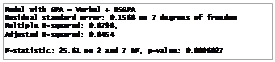Statistical significance does not necessarily imply practical significance

Adding variables always makes R2 increase, but this increase may not be significantPartial F Test

#### • Define two models:

Complete – uses all independent variables

 c

R2

Reduced – uses fewer independent variables

 r

R2

#### • Test the hypothesis that the unneeded variables do not contribute

Ho: b2 = 0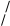Ha: b2 0

### Multicollinearity

#### • In multiple regression models, it is desirable for each independent variable to be highly correlated with Y, but it is not desirable for the X’s to be highly correlated with each other

This causes problems, and ultimately leads us to use various procedures to pick which variables to include and which to exclude from the model

#### • Teststatistic

(R2 - R2 ) u

F =     c                r              1 =

(1- R2 ) u

(0.894 - 0.880) /1(1- 0.894) / 3

= 0.396

c              2

Where n1 = number of b ‘s in Ho and n2 = n – 1 – (number of X’s in complete model)

For our example F0.05, 1, 3= 10.13, so fail to reject Ho

###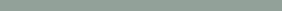Example

##### •  Salary Age Experience375233254721323814202533044184255302236827401523327345027   Suppose we have data on teacher’s salaries, their years of experience, and their age

One would expect that salaries would increase with both years of experience and age

Experience and age are also probably highly correlatedR Output with Age as Independent Variable

#### • Y = 2.291 + 0.642(X1)+ε

Call: lm(formula = Salary ~ Age, data = TeachSalary) Residuals:

Min 1Q Median 3Q Max

-7.475 -0.872 -0.122 1.568 5.305

Coefficients:

Estimate Std. Error t value Pr(>|t|) (Intercept) 2.2914    5.8622 0.391 0.70610

Age          0.6422    0.1368       4.694 0.00155 **

---

Signif. codes: 0 ‘***’ 0.001 ‘**’ 0.01 ‘*’ 0.05 ‘.’ 0.1 ‘ ’ 1 Residual standard error: 3.886 on 8 degrees of freedom Multiple R-squared: 0.7337,

F-statistic: 22.04 on 1 and 8 DF, p-value: 0.001552

R Output with Experience as Independent Variable

#### • Y=18.303+0.619(X2)+ε

Call: lm(formula = Salary ~ Experience, data = TeachSalary) Residuals:

Min       1Q Median 3Q Max

-6.3050 -1.1972 -0.3755 0.5050 5.1228

Coefficients:

Estimate Std. Error t value Pr(>|t|) (Intercept) 18.3033    2.3016    7.953   4.56e-05 ***

Experience   0.6191    0.1147    5.398   0.000648 ***

---

Signif. codes: 0 ‘***’ 0.001 ‘**’ 0.01 ‘*’ 0.05 ‘.’ 0.1 ‘ ’ 1 Residual standard error: 3.495 on 8 degrees of freedom Multiple R-squared: 0.7846,

F-statistic: 29.13 on 1 and 8 DF, p-value: 0.0006479

#### • Y=19.188-0.034(X1)+0.650(X2)+ε

Call: lm(formula = Salary ~ Age + Experience, data = TeachSalary) Residuals:

Min     1Q      Median 3Q Max

-6.2361 -1.1296 -0.4307 0.5467 5.1870

Coefficients:

Estimate Std. Error t value Pr(>|t|) (Intercept) 19.18821 14.28017    1.344       0.221

Age          -0.03406  0.54137   -0.063     0.952

Experience   0.64993  0.50471    1.288     0.239

Residual standard error: 3.735 on 7 degrees of freedom Multiple R-squared: 0.7847,

F-statistic: 12.75 on 2 and 7 DF, p-value: 0.004632Strange Happenings

###### • Coefficient of Age is -0.034

Indicates that older teachers tend to make less

###### •  Model with age as the only independent variable states that there is

a strong positive relationship between age and salary

###### • Confidence intervals for the independent variable coefficients include 0

They may even be positive or negative

###### • T-values for independent variables are very small

Age: t = -0.063, sig = 0.952

Experience: t = 1.288, sig = 0.239

###### • Model with both age and experience has same R2 as model with experience alone, and std. error of the estimate is slightly larger

Correlations

cor(TeachSalary)

Salary      Age    Experience

Salary       1.0000000 0.8565467 0.8857531

Age          0.8565467 1.0000000 0.9700520

Experience  0.8857531 0.9700520 1.0000000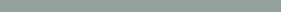Implications

###### •  Small t values are due to the fact that age and experience are

strongly correlated

Each t value describes the contribution of that particular independent variable after all other independent variables have been included in the model

Age contributes very little to the model when experience is already included and vice versa

###### •  You should always examine the pairwise correlations between all variables, including the dependent variable

Perfect multicollinearity exists when a variable is a sum of other variables

Collinearity Statistics

R provides an indicator of multicollinearity problems

Variance Inflation Factor (VIF)

Rule of thumb is that if it is greater than ~ 5 (your book says 10), there may be multicollinearity problems

You can also calculate Tolerance – the amount of variance in an independent variable that is not explained by the other independent variables

Tolerance – 1 – r2, where r2 is associated with the regression on all the other independent variables

Low tolerance (< 0.2) indicates multicollinearity problems

You cannot have a model with GRE verbal, quantitative, and total

scores as independent variables

>vif(TeacherBucks3)

Age     Experience

16.94939    16.94939

>1/vif(TeacherBucks3)

Age     Experience 0.05899916 0.05899916

### Other Collinearity Statistics

###### • Partial Correlation

The correlation that remains between two variables after removing the correlation that is due to their mutual association with the other variables. The correlation between the dependent variable and an independent variable when the linear effects of the other independent variables in the model have been removed from both.

###### • Part or Semipartial Correlation

The correlation between the dependent variable and an independent variable when the linear effects of the other independent variables in the model have been removed from the independent variable only. It is related to the change in R squared when a variable is added to an equation.

## Assignment Description

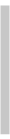Regression Analysis

 Regression

Correlation coefficient gives the strength of association

 Regression gives a mathematical function of the relationship

 Can be used to predict Y from knowledge of X



 Types of Variables

Dependent

 Also known as response or endogenous variables

 Y1, Y2, …,Yr

 Independent

 Also known as predictoror exogenous

 X1, X2, …, Xr

 The finding of a “statistically significant” association in a particular study does not establish a causal relationship

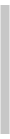Cause and Effect

To evaluate claims of causality, the investigator must consider criteria that are external to the specific characteristics and results of a particular study

 A functional relation between two variables X and Y is expressed by

###  Specify the variables in the model and the exact form of the relationship between them

 Collect data



 Steps in the Regression Model Building Procedure

 Functional and Statistical Relations

Y = f(X)

 A statistical relation is not necessarily perfect

 Some, but not all of the variation in the dependent variable can be predicted by the independent variable

###  Estimate the parameters of the model

 Statistically test the utility of the developed model and check whether the assumptions of the simple linear regression model are satisfied

 Use the model for prediction

#####  Assumptions of Linear Regression  The true (population) regression line of Y as a linear function of X

is Yi = a + bXi + ei

#####  For the ith level of the independent Xi the expected value of the error component is equal to zero

 The variance of the error component ei is constant for all levels of X

 Homoscedasticity

#####  The values of the error component for any two ei are pairwise uncorrelated

 The error components are normally distributed

 Required for construction of hypothesis tests and confidence intervals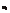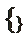### 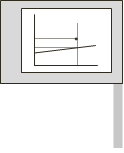Fitting Criteria  Y^i  P(Xi, Yi)  Yi  We want to predict Y, so we want to minimize deviation in Y from the line

####  i  Pick a straight line that has minimum vertical deviations

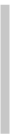Our estimated Y is

Yˆ = a + bX i

###  i  The residual error is ei=(Yi-Yˆ)

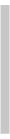Least Squares Solution

 Least Squares Criterion

Requires the following “normal” equations be satisfied

 Best fit is not simply     n                                                                                                                                                                                                                                                                                                               n                       n

 i

min å(Yi  - Yˆ )

na + bå Xi   = åYi

i=1

i=1

i=1

 There is not a unique solution to this

aå + bå X 2= å X Y

 (+ and - errors cancel)

 Least Squares criterion gives a unique solution

i

 n                          n                          n

i=1

i=1

i i

i=1

 i i

min ån    (Y  - Yˆ )2

i=1 Since a = Y - bX we can determine the intercept a once we know b

####  The best fit line also passes through (X,Y)

n                  n           n

nå XiYi  - å Xi åYi b =    i=1            i=1                    i=1

 å  2       å

n              æ  n         ö2

n    X  - ç     X ÷ Sum of squared deviations won’t work because it depends on scale of X and Y and the number of observations

 To compare how regressions for different data sets compare, we

i

i=1

è i=1       ø

can use

 Simple correlation coefficient r

 Coefficient of determination r2

 Standard error of the estimate sy.x

 Finding the Slope of the Regression Coefficient b

 Assessing Goodness of Fita = Y - bX

###  Texas Hair Height Example  Correlation Coefficient r  Pearson’s r is a dimensionless measure of the degree of linear association between two variables X and Y

####  Ranges from -1 < r < +1

ån    X Y  -(ån    X  )(å Y ) / n

####  Pearson’s r is:

17295 − (80)(2000)/10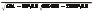𝑟 =

= 0.9638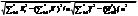Xi Yi Xi^2 Yi^2 XiYi 4.5 100 20.25 10000 450 6.0 130 36.00 16900 780 5.5 160 30.25 25600 880 7.0 180 49.00 32400 1260 7.5 190 56.25 36100 1425 8.0 200 64.00 40000 1600 10.0 220 100.00 48400 2200 9.0 240 81.00 57600 2160 10.5 280 110.25 78400 2940 12.0 300 144.00 90000 3600 80 2000 691 435400 17295

r =                 i=1 i i

i=1 i

i=1 i

#### 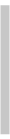Standard deviations are

 There is a direct relationship between r and our

 Sx= 62.716 Sy= 2.380

### computation of b

b = r Sy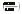S

𝑏 = 0.9638

=25.392

x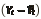Total Variation

 Coefficient of Determination r2

𝑌𝑖   𝑌  =                     + (𝑌𝑖   𝑌)



 i

Residual error ei = (Yi - Yˆ ) provides useful information on the fit of the regression line

 First divide variation of Y about its mean

###  First term on right hand side is residual error, second term is the difference between predicted Y and mean of Y

into two parts:

n(Y - Y )2

####  Total Sum of Squares = Error Sum of Squares plus

å i

i=1

 Variation explained by regression

 Residual variation not explained by regression

#### Regression Sum of Squares

 TSS = ESS + RSS

 Expand this to yieldån   (Y  - Y )2  = ån    (Y  - Yˆ )2  + ån    (Yˆ - Y )2

i

i=1

i             i

i=1

i

i=1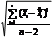Standard Error of Estimate

 Coefficient ofDetermination r2

r 2 = 1- ESS = RSS

TSS TSS

SY × X =

 0 < r2 < 1

####  Generally high r2 indicates a good fit

 This is a statistical explanation of variation, not necessarily a causal explanation

####  r2 can be artificially inflated in spatial and time series studies

 Standard deviation of the residuals about the regression line

 Also called Root Mean Squared Error (RMSE)

###  Provides a numerical value of the error we are likely to make when utilizing X to predict Y

Inferences on the Slope of the Regression Line

 Interpreting Std. Error of the Estimate

We are often interested in determining the sensitivity of Y to changes in X

 If we assume  the errors about the regression  line are normally                                                                                                                                                                                                                                s                                 S

distibuted, we can estimate that 95% of the estimates will be + 2

standard errors

 For our Income vs. Trips example this equates to about 3 trips per

s  = @ Y × X                                              = S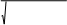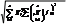b

 b

 å       å

n                   æ  n           ö2

X - ç     ÷  / nday per household

 In a city with 100,000 households, this would be roughly 300,000 trips

i

i=1

è i=1 ø

 The test statistic is

t = b - b

Sb

b - ta / 2,n-2 Sb £ b £ b + ta / 2,n-2 Sb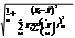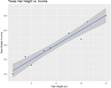Shapeof Confidence Intervals

 Confidence Interval for mY.X for a Given X

 0

SYˆ = SY × X

###  ^  The standard error of Yo increases with

####  Standard error of the estimate SY.X

 Reciprocal of the sum of squared deviations

 Sample size n Difference between the value X0 and the mean X Confidence interval is narrowest at X0 = X

 This is due to the impact of possible errors in both a and b

Regression in R

We run a regression analysis using the lm() function – lm stands for ‘linear model’. This function takes the general form:

newModel<-lm(outcome~ predictor(s), data = dataFrame, na.action = an action))

Regression in R

or we can tell R what dataframe to use (using data = nameOfDataFrame), and then specify the variables without the dataFrameName\$ before them:

albumSales.1 <- lm(sales ~ adverts, data = album1)

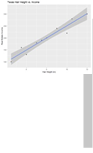Texas Hair Height

The relationship between height of hair and income from real estate is

approximately

 y = -3.137 + 25.392x

 > Hair <- lm(formula = Income ~ HairHgt,

   data = TexasHair)

 >summary(Hair)Min 1Q Median 3Q Max-30.784 -8.738 1.348 12.304 23.480 Real Estate Hair Height, in Income (\$ x 1000) Coefficients: 4.5 100 Estimate Std. Error t value Pr(>|t|) 6 130 (Intercept) -3.137 20.647 -0.152 0.883 5.5 160 HairHgt 25.392 2.484 10.223 7.2e-06 *** --- 7 180 Signif. codes: 0 ‘***’ 0.001 ‘**’ 0.01 ‘*’ 0.05 ‘.’ 0.1 ‘ ’ 1 7.5 190 Residual standard error: 17.74 on 8 degrees of freedom 8 200 Multiple R-squared: 0.9289, Adjusted R-squared: 0.92 10 220 F-statistic: 104.5 on 1 and 8 DF, p-value: 7.198e-06 9 240 10.5 280 12 300

Residuals:Is it free to get my assignment evaluated?

Yes. No hidden fees. You pay for the solution only, and all the explanations about how to run it are included in the price. It takes up to 24 hours to get a quote from an expert. In some cases, we can help you faster if an expert is available, but you should always order in advance to avoid the risks. You can place a new order here.

How much does it cost?

The cost depends on many factors: how far away the deadline is, how hard/big the task is, if it is code only or a report, etc. We try to give rough estimates here, but it is just for orientation (in USD):

 Regular homework \$20 - \$150 Advanced homework \$100 - \$300 Group project or a report \$200 - \$500 Mid-term or final project \$200 - \$800 Live exam help \$100 - \$300 Full thesis \$1000 - \$3000

How do I pay?

Credit card or PayPal. You don't need to create/have a Payal account in order to pay by a credit card. Paypal offers you "buyer's protection" in case of any issues.

Why do I need to pay in advance?

We have no way to request money after we send you the solution. PayPal works as a middleman, which protects you in case of any disputes, so you should feel safe paying using PayPal.

Do you do essays?

No, unless it is a data analysis essay or report. This is because essays are very personal and it is easy to see when they are written by another person. This is not the case with math and programming.

Why there are no discounts?

It is because we don't want to lie - in such services no discount can be set in advance because we set the price knowing that there is a discount. For example, if we wanted to ask for \$100, we could tell that the price is \$200 and because you are special, we can do a 50% discount. It is the way all scam websites operate. We set honest prices instead, so there is no need for fake discounts.

Do you do live tutoring?

No, it is simply not how we operate. How often do you meet a great programmer who is also a great speaker? Rarely. It is why we encourage our experts to write down explanations instead of having a live call. It is often enough to get you started - analyzing and running the solutions is a big part of learning.

What happens if I am not satisfied with the solution?

Another expert will review the task, and if your claim is reasonable - we refund the payment and often block the freelancer from our platform. Because we are so harsh with our experts - the ones working with us are very trustworthy to deliver high-quality assignment solutions on time.

## Popular Solved Assignments Like This

Customer Feedback

"Thanks for explanations after the assignment was already completed... Emily is such a nice tutor! "

Order #13073